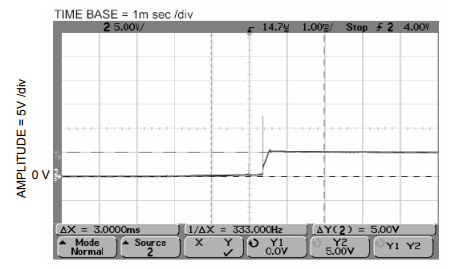Select Page

## Introduction

Shorting of the DC voltage terminal of a MMIC amplifier to ground while DC power is connected can cause permanent damage to the amplifier. This can occur, for example, in a test setup where the amplifier is in a fixture with clip leads for the DC connections; the clips are accidentally moved and they momentarily short the DC and ground terminals together.

## Cause of Failure

The short-circuit current is determined by the current-limit setting of the power supply. The abrupt cessation of current, when the short is removed, causes an inductive voltage kick. A few feet of clip lead can have typically a uH of inductance, which resonates with the bypass capacitor at the amplifier DC terminal. For a 1000 pF capacitor the resonance is in the range of a few MHz. The transient tends to exceed the absolute maximum voltage rating of the amplifier.

## Evaluation

The bias circuit in Figure 1 was used to evaluate the effect of momentarily shorting the DC supply terminal to ground. Amplifier “A” is MNA-4A. F1 is a surface-mount ceramic-ferrite EMI filter having 13 and 30 dB attenuation at 10 and 20 MHz respectively. D1 is a 1N755A zener diode, found effective in preventing damage due to momentary shorting. Testing was done with and without the zener in two circuit configurations: using F1 as shown, and replacing it with a wire jumper.

Figure 2 illustrates the effect of removing a short-to-ground that was applied at the DC input to model MNA-4. The bias circuit in Figure 1 was used, including the EMI filter. Voltage was +5V, and the current limit was set to 4A. The oscilloscope was connected at Point B in Figure 1. A spike occurs at the beginning of the ramp whereby the power supply restores the normal voltage.

Figure 3 shows the same occurrence, but with greatly expanded time scale. The spike is revealed to have a peak of about 19V, exceeding the 7V absolute maximum voltage rating. The device failed as a result of this test.Figure 2: Voltage transient when short is removed, 5V supply; with EMI filter.Figure 3: Same as Figure 1 with expanded time scale.

Figure 4 was obtained under the same conditions as for Figure 3, except that a wire jumper replaced the EMI filter. The shape of the transient is different, but the 16V amplitude similarly exceeded the 7V rating by a large factor. The device failed as a result of this test.Figure 4: Voltage transient when short is removed, 5V supply; without EMI filter.

## Prevention

No damage occurred when devices were tested for shorting survival with a 7.5V ±5% zener diode (1N755A) connected between the MNA-4 DC terminal and ground. This value was chosen so that the zener will not conduct when applying voltage up to the absolute maximum rating of the amplifier. Both configurations were tested: with the EMI filter (Figures 5 and 6) and with a jumper replacing it (Figures 7 and 8). The DC supply was set at 5V for the traces in Figures 5 and 7; it was set at 7V for Figures 6 and 8.

The oscilloscope traces show that the zener diode clamps Point B to a voltage in the range 7.7 to 8.6V. An initial peak of 11.7V occurs when the EMI filter is used, but is absent with the jumper.

The supply was current-limited at 5A. Very similar results were obtained using 1A and 3A current limiting.